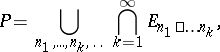# A-operation

(diff) ← Older revision | Latest revision (diff) | Newer revision → (diff)

operationA set-theoretical operation, discovered by P.S. Aleksandrov  (see also , ). Letbe a system of sets indexed by all finite sequences of natural numbers. The setwhere the union is over all infinite sequences of natural numbers, is called the result of the-operation applied to the system.

The use of the-operation for the system of intervals of the number line gives sets (called-sets in honour of Aleksandrov) which need not be Borel sets (see-set; Descriptive set theory). The-operation is stronger than the operation of countable union and countable intersection, and is idempotent. With respect to-operations, the Baire property (of subsets of an arbitrary topological space) and the property of being Lebesgue measurable are invariant.

How to Cite This Entry:
A-operation. Encyclopedia of Mathematics. URL: http://encyclopediaofmath.org/index.php?title=A-operation&oldid=16633
This article was adapted from an original article by A.G. El'kin (originator), which appeared in Encyclopedia of Mathematics - ISBN 1402006098. See original article